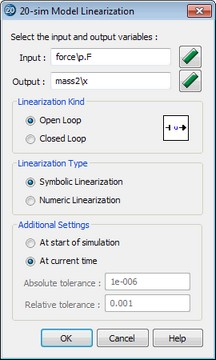﻿ 20-sim webhelp > Toolboxes > Frequency Domain Toolbox > Model Linearization > Linearization Tolerances

# Linearization Tolerances

Navigation:  Toolboxes > Frequency Domain Toolbox > Model Linearization >

# Linearization TolerancesWhen using numerical linearization, absolute and relative tolerances can be set. In this section is explained how 20-sim uses these tolerances to derive a linear system for the model equations.## Algorithm

Suppose you have chosen the following tolerance values.

alpha = absolute tolerance (e.g. 1e-6)

beta = relative tolerance (e.g. 1e-3)

Suppose we have the following model:

ddt (x) = 4 * x + 2 * u; // with u = 1

y = 1 * x + 3 * u; // with x = 2

During the linearization procedure (suppose linearization from u to y ) 20-sim will vary the input variable and state variables. First we will show the variation for the input u:

u' = (1 + beta)*u + alpha

u'' = (1 - beta)*u - alpha

delta_u = beta*u + alpha

this will yield

x_dot' = 10.002002 and y' = 5.003003

x_dot'' = 9.997998 en y'' = 4.996997

Out of this 20-sim will calculate the B and D vectors of the state-space ABCD representation:

B = (x_dot' - x_dot'' ) / (2 * delta_u) = 2

D = (y' - y'') / (2 * delta) = 3

The variation of state variables is done accordingly:

x' = (1 + beta)*x + alpha

x'' = (1 - beta)*x - alpha

delta_x = beta*x + alpha

this will yield

x_dot' = 10.008004 en y' = 5.002001

x_dot'' = 9.991996 en y'' = 4.997999

Out of this 20-sim will calculate the A matrix and C vector of the state-space ABCD representation:

A = (x_dot' - x_dot'') / 2*delta_x = 4

C = (y' - y'') / 2*delta_x = 1

The example system is linear, so the corresponding ABCD representation will give an equal system. The shown algorithm works equivalent for non-linear models. Suppose we have the following non-linear model:

ddt (x) = 4 * sin(x) + 2 * u; // with u = 1

y = 1 * x + 3 * u; // with x = 2

This will yield:

B = 2, D = 3, A = -1.668445, C = 1;

## Tolerance Values

The absolute tolerance is necessary only if the input or state is zero. In that case the contribution of the relative tolerance is zero an will not give a contribution to the algorithm. There is however a problem with the absolute tolerance. Suppose the input = 1e-12 and the state = 1e+6 (ill-scaled model). For the input an absolute tolerance of 1e-6 will yield a far too large deviation, while the same absolute tolerance is negligible compared to the state. Only if both the state and input are nonzero the absolute tolerance can be made non-zero to yield good results.